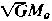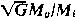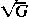Also found in: Dictionary.

See gravitational waves.
Collins Dictionary of Astronomy © Market House Books Ltd, 2006
The following article is from The Great Soviet Encyclopedia (1979). It might be outdated or ideologically biased.

the radiation of gravitational waves, or waves of attraction, by nonuniformly moving masses (bodies).

The existence of gravitational waves stems from A. Einstein’s general theory of relativity (the theory of attraction), which he formulated in 1916. The equations for the gravitational field are mathematically very complicated and have been solved only for a weak field. The solution corresponds to transverse waves that are propagating at the speed of light in a vacuum. As yet, however, gravitational waves have not been reliably detected because of their extremely low intensity and exceedingly weak interaction with matter. Although the overwhelming majority of physicists are convinced of their existence, experimentation must provide a final answer to the question of whether gravitational radiation exists.

There is considerable analogy between the laws of the interaction of electrical charges and the gravitational interaction of masses. For instance, Coulomb’s law is similar to Newton’s law of universal gravitation, and Maxwell’s equations of electrodynamics are similar to Einstein’s equations for a weak gravitational field. Therefore, the laws of gravitational radiation are in form also very close to the laws governing the radiation of electromagnetic waves. Accelerating electrical charges are the source of electromagnetic waves, and the greater the charge and its acceleration, the more powerful will be the electromagnetic radiation. Similarly, any body moving with acceleration may be a source of gravitational radiation. The role of the “gravitational charge,” which creates a field of attraction, is played here by the gravitational mass of the body Mg or more accurately (in order to obtain the dimension of charge), by the quantity. where G is the constant of gravitation found both in the law of universal attraction and Einstein’s equations. Upon nonuniform motion of the mass, the gravitational field may be separated from the mass that created it and may propagate independently in the form of gravitational waves.

The strength of gravitational radiation, in complete analogy to electrodynamics, is determined by the magnitude of the gravitational charge and by its acceleration but is very small indeed. The reason for this lies primarily in the small-ness of the constant of gravitation G, which determines the “force” of gravitational interaction. Of all known types of interaction, gravitational interaction is the weakest. For example, for two electrons it is 1042 times weaker than their electromagnetic interaction. Moreover, in contrast to electrical charges all gravitational charges (gravitational masses) have the same sign, and the specific gravitational charge—the ratio of the gravitational charge to the inert mass of the body.,—is the same for all bodies and is equal to(since it follows from experiment that the gravitational mass, upon normal selection of the gravitational constant, is strictly equal to the inert mass). Therefore, by analogy with the electromagnetic radiation of a system of electrical charges of like sign having the same specific charge, the gravitational radiation of some parts of the body moving under acceleration will necessarily be partially compensated for by the radiation of other parts of the body (incomplete compensation occurs only when there is some distance between the individual parts of the radiating mass). Such radiation, like the radiator itself, is said to be quadrupole. Thus, the variable motion of some mass may lead only to quadrupole radiation of gravitational waves of very low intensity.

Gravitational wave receivers—gravitational antennas, which must also be of the quadrupole type—are also ineffective. Any pair of masses or an extended body and a sensitive device that records small relative displacements of masses may serve as a gravitational antenna. A gravitational wave creates a variable acceleration field that propagates at the speed of light c. The amplitude of this field declines in inverse proportion to the distance from the radiator. The two masses of the gravitational antenna, which are located at some distance from each other in this acceleration field, will oscillate with respect to each other at the frequency of radiation. The low magnitude of the relative displacement of the masses makes the detection of gravitational radiation difficult.

The power of the gravitational radiation that can be produced under laboratory conditions from a transmitter (generator) of real dimensions is extremely low (of the order of 10−20 watts [W]). Therefore, attempts are being made to detect the gravitational radiation from sources of nonterres-trial origin. The most reliable of these (continuous sources) are nearby massive binary stars with a relatively short rotation period (1.5–4 hours) and components having masses of the order of the mass of the sun (for example, the binary star WZ in Sagittarius). The power of the gravitational radiation of such stars is ∼1023 W. which corresponds to a surface density of the gravitational radiation flux near the earth of the order of 10−13 W/m2. A greater flux density (10−4-104 W/m2) may be anticipated for some explosive processes in stars.

In 1966, counting on such bursts of gravitational radiation of extraterrestrial origin, the American physicist J. Weber devised a gravitational radiation receiver in which an aluminum cylinder 1.5 m long and weighing 1.5 tons served as the gravitational antenna. The cylinder was suspended on fine threads from a frame consisting of steel blocks sandwiched with rubber packing (as an antiseismic filter). The cylinder and frame were placed in a vacuum chamber, and the entire unit was situated far from industrial interference.

Quartz piezoelectric pickups attached along the cylinder transform mechanical vibrations into electrical signals. A sensitive amplifier (in which the input circuit is cooled to the temperature of liquid helium to reduce thermal oscillations) makes it possible to record the mechanical vibrations of the cylinder that correspond to the movement of one end of the cylinder by 2 x 10 −14 cm with respect to the other. A second cylinder having the same frequency characteristics is located at a distance of about 1,000 km from the first. Piezoelectric pickups are also attached to it. The electrical signals from both cylinders enter a coincidence circuit so that the bursts of gravitational radiation, which should synchronously give rise to vibrations in both cylinders, can be distinguished from bursts of thermal oscillations, which are not correlated—that is, do not coincide in time. The coincidence circuit produces an output pulse if the signals exceed some selected threshold level and if they are appropriately shifted in time.

The device operated for a prolonged period of time and resulted in the detection of several dozen coincident bursts that exceeded the noise level by a factor of approximately 10. It is possible that the joint excitation of both cylinders by gravitational waves from some common source was observed. However, the flux density of the gravitational radiation corresponding to the recorded bursts was several tens of thousands of watts per sq m, which is quite a large quantity for the most likely distances to the explosive sources of extraterrestrial origin. Further experimental research should confirm or refute the results obtained by Weber. The sensitivity of Weber’s unit was not very great (104 W/m2), but it is not the maximum obtainable.

The detection of gravitational radiation from extraterrestrial sources would open up a new channel of information on physical processes in the cosmos.

### REFERENCES

Weber, J. Obshchaia teoriia otnositel’nosti i gravitatsionnye volny. Moscow, 1962. (Translated from English.)
Braginskii, V. B. “Gravitatsionnye volny i popytki ikh obnaruzheniia.” Zemlia i Vselennaia, 1965, no. 5.
Braginskii, V. B. “Gravitatsionnoe izluchenie i perspektivy ego eksperimental’nogo obnaruzheniia.” Uspekhi fizicheskikh nauk, 1965, vol. 86, part 3, pp. 433–46.
Braginskii, V. B., and V. N. Rudenko. “Relativistskie gravitatsionnye eksperimenty.” Uspekhi fizicheskikh nauk, 1970, vol. 100, part 3, p. 395.
Braginskii, V. B. Fizicheskie eksperimenty s probnymi telami. Moscow, 1970. Chapter 3.

V. B. BRAGINSKII

[‚grav·ə′tā·shən·əl ‚rād·ē′ā·shən]
(relativity)
McGraw-Hill Dictionary of Scientific & Technical Terms, 6E, Copyright © 2003 by The McGraw-Hill Companies, Inc.
References in periodicals archive ?
One consequence of this conformally flat approximation to the three-metric is that the emission of gravitational radiation is not explicitly evolved.
Penrose, "Errata: an approach to gravitational radiation by a method of spin coefficients, " Journal of Mathematical Physics, vol.
LIGO was first planned during the mid-1970s, it was first funded during the 1980s, its construction started during the 1990s, it was first turned on during the 2000s, and its advanced version - the LIGO that first heard gravitational radiation - was just turned on last September.
They cover a brief review of general relativity, gravitational waves, beyond the Newtonian limit, sources of gravitational radiation, gravitational-wave detectors, analyzing gravitational-wave data, and gravitational-wave astronomy and astrophysics.
During the merger, directional emission of gravitational radiation would cause the black hole to recoil.
"We hope the telescope will have the power and sensitivity to detect gravitational radiation that Einstein says is produced by anything that moves and accelerates.
The vibrational displacement caused by a single quantum of gravitational radiation of angular frequency [omega] = 2[pi][v.sub.0] can easily be computed by direct substitution of these values in Eq.
These binaries have extremely short orbital periods (shorter than about one hour), and are predicted to be strong sources of gravitational radiation, strong enough to be detected with the Laser Interferometer Space Antenna.
According to Einstein's theory, such a system might be producing an effect called gravitational radiation, noted Jon Miller of the University of Michigan in Ann Arbor.lthough cosmologists have yet to see the effect directly, they think gravitational radiation propagates across the universe like waves on the surface of a puddle and remains unchanged as it travels.
Searching for Gravitational Radiation "The most stringent limits so far on the amount of such radiation were announced in the February 15, 1983, AstrophysicalJournal Letters.
The kick is believed to be generated when two giant black holes from different galaxies merge, unleashing an enormous burst of gravitational radiation. These proposed ripples in space-time, predicted by Einstein's theory, travel at the speed of light.

Site: Follow: Share:
Open / Close